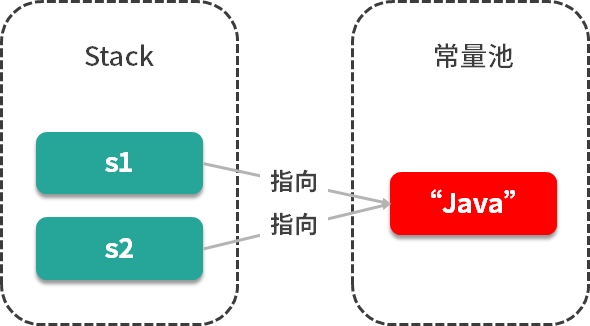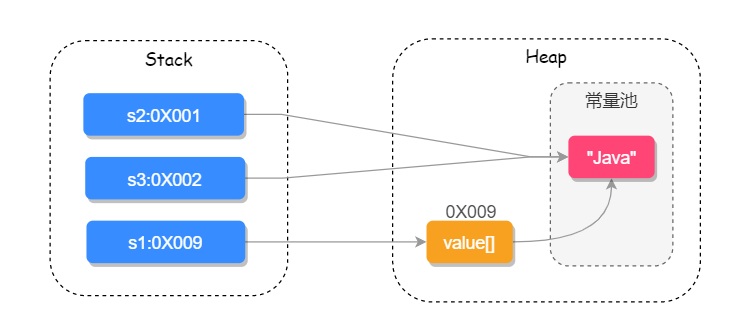# 重新学习java中的String

## 首先先抛出一个问题：String是如何实现的？它有哪些重要的方法？

### 典型回答

	public final class String implements
java.io.Serializable,Comparable<String>,CharSquence{
//用于存储字符串的值
private final char value[];
//缓存字符串的  hash code
private int hash;  //默认为 0
//以下为其他内容
.....
}


String源码中包含以下几个重要的方法

### 1.多构造方法

String字符串有以下4个重要的构造方法：

	//String 为参数的构造方法
public String (String original){
this.value = original.value;
this.hash = original.value;
}
//char[] 为参数的构造方法
public String (char value[]){
this.value = Arrays.copyOf(value,value.length);
}
//StringBuffer 为参数的构造方法
public String (StringBuffer buffer){
synchronized(buffer){
this.value = Arrarys.copyOf(buffer.getValue(),buffer.length());
}
}
//StringBuilder 为参数的构造方法
public String (StringBuilder builder){
this.value = Arrarys.copyOf(builder.getValue(),builder.length());
}


### 2.equals()比较两个字符串是否相等

	public boolean equals(Object anObject){
//对象引用相同直接返回 true
if(this == anObject){
return true;
}
//判断需要对比的值是否为 String 类型,如果不是则直接返回 false
if(anObject instanceof String){
String anotherString = (String)anObject;
int n = value.length;
//判断传入的值与对比值的长度相等就执行,如果不相等则直接返回 false
if(n == anotherString.value.length){
//把两个字符串都转换为  char  数组对比
char v1[] = value;
char v2[] = anotherString.value;
int i = 0;
//循环对比两个字符串的每一个字符
while(n-- != 0){
//如果其中有一个字符不相等就返回 false,否则就继续对比
if (v1[i] != v2[i])
return false;
i++;
}
return true;
}
}
return false;
}


String类型重写了Object中的equals()方法,equals()方法需要传递一个Object类型的参数值，在比较时会先通过instanceof判断是否为String类型，如果不是则会直接返回false,instanceof的使用如下：

	Object oString = "123";
Object oInt = 123;
System.out.println(oString instanceof String); // 返回  true
System.out.println(oInt instanceof String);// 返回 false


### 3.compareTo() 比较两个字符串

compareTo() 方法用于比较两个字符串，返回的结果为 int 类型的值，源码如下：

public int compareTo(String anotherString){
int len1 = value.length;
int len2 = anotherString.value.length;
// 获取到两个字符串长度最短的那个 int 值
int lim = Math.min(len1, len2);
char v1[] = value;
char v2[] = anotherString.value;
int k = 0;
// 对比每一个字符
while (k < lim) {
char c1 = v1[k];
char c2 = v2[k];
if (c1 != c2) {
// 有字符不相等就返回差值
return c1 - c2;
}
k++;
}
return len1 - len2;
}


• equals() 可以接收一个Object类型的参数，而 compareTo() 只能接收一个String类型的参数；
• equals() 返回值为Boolean，而compareTo() 的返回值则为 int 。

### 4.其他重要方法

• indexOf() ：查询字符串首次出现的下标位置
String s = "hello";
int i1 = s.indexOf('h'); // i1=0
int i2 = s.indexOf('o');//	i2=4
int i3 = s.indexOf('a');//	i3=-1
int i4 = s.indexOf('l');//	i4=2

• lastIndexOf() ：查询字符串最后出现的下标位置
String s = "hello";
int i1 = s.lastIndexOf('h'); // i1=0
int i2 = s.lastIndexOf('o');//	i2=4
int i3 = s.lastIndexOf('a');//	i3=-1
int i4 = s.lastIndexOf('l');//	i4=3

• contains() ： 查询字符串中是否包含另一个字符串
String s = "hello";
Boolean b1 = s.contains("llo"); // b1=true
Boolean b2= s.contains('halo');//	b2=false

• toLowerCase() ：把字符串全部转换成小写
String s = "Hello";
String s1 = s.toLowerCase(); // s1=hello

• toUpperCase()：把字符串全部转换成大写
String s = "Hello";
String s1 = s.toUpperCase(); // s1=HELLO

• length()：查询字符串的长度
String s = "Hello";
int i1= s.length(); // i1=5

• trim()：去掉字符串首尾空格
String s = " H e llo ";
String s1= s.trim(); // s1=H e llo

• replace()：替换字符串中的某些字符
String s = " H e llo ";
String s1= s.replace(' ',','); // s1=,H,e,llo,
String s2= s.replace(" ",""); // s1=Hello

• split()：把字符串分割并返回字符串数组
String s = " H e llo ";
String []arrayS1= s.split(" "); // arrayS1=[,H,e,llo]

• join()：把字符串数组转为字符串
String s = " H e llo ";
String []arrayS1= s.split(" "); // arrayS1=[,H,e,llo]
String s1= String.join("",arrayS1); // 此时,第一个参数为拼接格式,第二个参数为字符串数组 s1=Hello
String s2= String.join("-",arrayS1); //  s2=-H-e-llo


### 考点分析

String源码属于所有源码中最基础、最简单的一个，对String源码的理解，也反应了我们的Java基础功底。

String问题如果再延伸一下，会问到一些更多的知识细节，这也是大厂一贯使用的面试策略，从一个知识点入手然后扩充更多的知识细节，对于String也不例外，通常还会关联的询问以下问题：

• 为什么String类型要用final修饰？
• ==和equals的区别是什么？
• String和StringBuilder、StringBuffer有什么区别？
• String的intern()方法有什么含义？
• String类型在JVM(Java虚拟机)中是如何存储的？编译器对String做了哪些优化？

### 知识扩展

#### 1.为什么String类型要用final修饰？

public final class String implements java.io.Sericlizable, Comparacle<String>, CharSequence { // ....}


Java语言之父 James Gosling 的回答是，他会更倾向于使用final，因为它能够缓存结果，当你在传参时，不需要考虑谁会修改它的值；如果是可变类的话，则有可能需要重新拷贝出来一个新值进行传参，这样在性能上就会有一定的损失。

James Gosling 还说迫使String类设计成不可变的另一个原因是安全，当你在调用其他方法时，比如调用一些系统级指令之前，可能会有一系列校验，如果是可变类的话，可能在你校验过后，它内部的值有被改变了，这样有可能会引起严重的系统崩溃问题，这样迫使String类设计成不可变类的一个重要原因。

String s1 = "java";
String s2 = "java";#### 2.==和equals的区别是什么？

==对于基本数据类型来说，是用于比较 “值” 是否相等的；而对于引用类型来说，是用于比较引用地址是否相同的。

public boolean equals(Object obj){
return (this == obj);
}


public boolean equals(Object anObject){
//对象引用相同直接返回  true
if(this == anObject){
return true;
}
//判断需要对比的值是否为 String  类型，如果不是则直接返回 false
if (anObject instanceof String) {
String anotherString = (String)anObject;
int n = value.length;
if(n == anotherString.value.length){
// 把两个字符串都转换为 char 数组对比
char v1[] = value;
char v2[] = anotherString.value;
int i = 0;
//村换对比两个字符串的每一个字符
while(n-- != 0){
//如果其中有一个字符不相等就返回 false,否则继续对比
if(v1[i] != v2[i])
return false;
i++;
}
return true;
}
}
return false;
}


#### 3.String和StringBuilder、StringBuffer有什么区别？

@Override
public synchronized StringBuffer append(Object obj){
toStringCache = null;
super.append(String.valueOf(obj));
return this;
}
@Override
public synchronized StringBuffer append(String str){
toStringCache = null;
super.append(str);
return this;
}


#### 4.String的intern()方法有什么含义？

intern()方法的意思是：如果该字符串不存在常量池中，将字符串放入常量池，并返回该字符串。如果该字符串存在常量池中，则直接返回该字符串。

    /**
* Returns a canonical representation for the string object.
* <p>
* A pool of strings, initially empty, is maintained privately by the
* class {@code String}.
* <p>
* When the intern method is invoked, if the pool already contains a
* string equal to this {@code String} object as determined by
* the {@link #equals(Object)} method, then the string from the pool is
* returned. Otherwise, this {@code String} object is added to the
* pool and a reference to this {@code String} object is returned.
* <p>
* It follows that for any two strings {@code s} and {@code t},
* {@code s.intern() == t.intern()} is {@code true}
* if and only if {@code s.equals(t)} is {@code true}.
* <p>
* All literal strings and string-valued constant expressions are
* interned. String literals are defined in section 3.10.5 of the
*
* @return  a string that has the same contents as this string, but is
*          guaranteed to be from a pool of unique strings.
*/
public native String intern();


#### 5.String 类型在 JVM（Java 虚拟机）中是如何存储的？编译器对 String 做了哪些优化？

String 常见的创建方式有两种，new String()的方式和直接赋值的方式，直接赋值的方式会先去字符串常量池中查找是否已经有此值，如果有则把引用地址直接指向此值，否则会先在常量池中创建，然后再把引用指向此值；而new String()的方式一定会先在堆上创建一个字符串对象，然后再去常量池中查询此字符串的值是否已经存在，如果不存在会先在常量池中创建此字符串，然后把引用的值指向此字符串，如下代码所示：

String s1 = new String("Java");
String s2 = s1.intern();
String s3 = "Java";
System.out.println(s1 == s2);// false
System.out.println(s2 == s3);// true小贴士：JDK1.7之后把永生代换成了元空间，把字符串常量池从方法区移到了Java堆上。

String s1 = "Ja" + "va";
String s2 = "Java";
System.out.println(s1 == s2);//true


Compiled from "StringTest.java"
public class com.qinzhouyue.interview.StringExample{
public com.qinzhouyue.interview.StringExample();
Code:
1: invokespecial #1  //Method java/lang/Object."<init>":()V
4: return
LineNumberTable:
line 3: 0
public static void main(java.lang.String[]);
Code:
0:	1dc		#2 		//String Java
2:	astore_1
3:	1dc		#2		//String Java
5:	astore_2
6:	getstatic	#3	//Field java/lang/System.out:Ljava/io/PrintStream;
11:	if_acmpne	18
14:	iconst_1
15:	goto		19
18:	iconst_0
19:	incokevirtual	#4		//Method java/io/PrintStream.println:(Z)V
22:	return
LineNumberTable:
line	5:	0
line	6:	3
line	7:	6
line	8:	22

}


### 小结

03-0711-1331
12-197293
08-164342
09-031万+
06-304759
08-274万+
05-1386
01-165万+